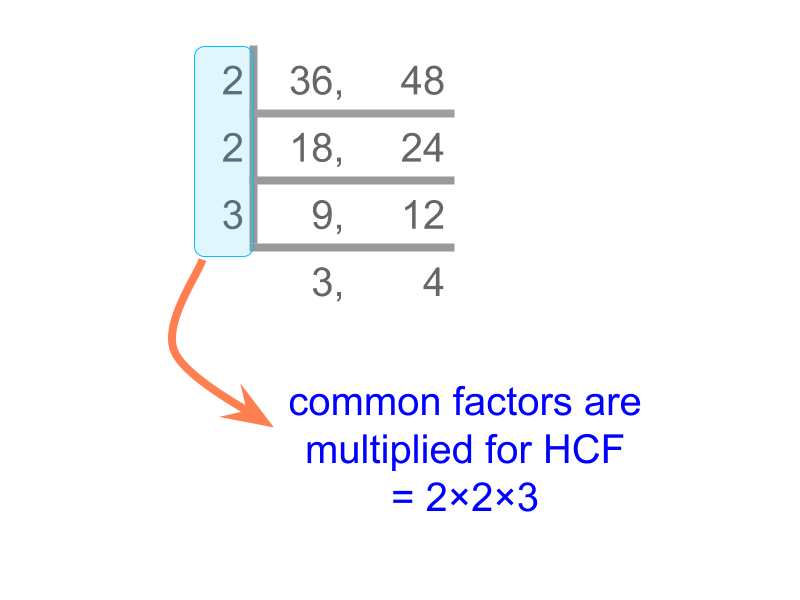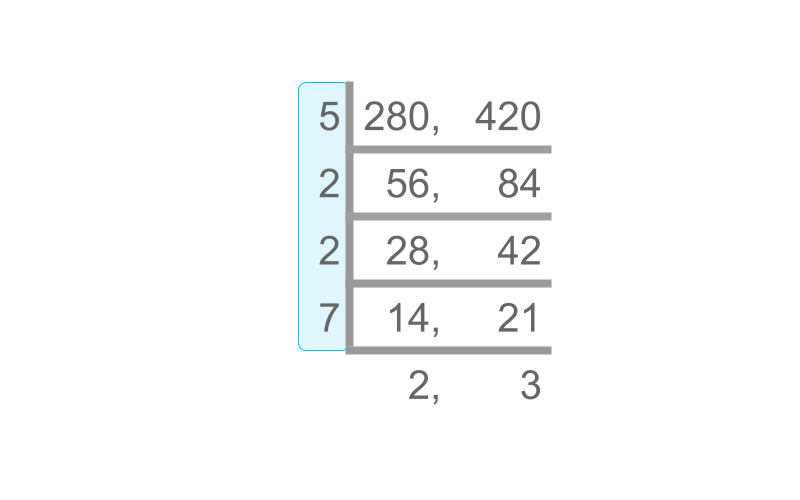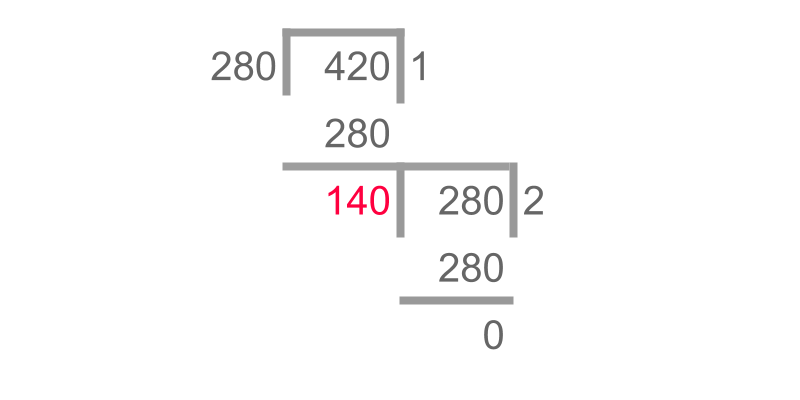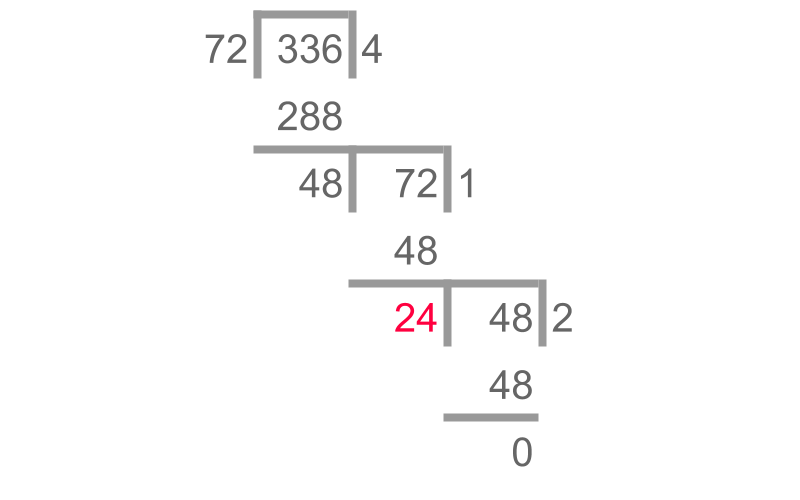maths > wholedivisors

Common Factors & HCF

what you'll learn...

overview

common factors of two or more numbers,

co-prime numbers,

highest common factor of two or more numbers,

highest common factor using factorization method,

highest common factor using division method

Common Factors

Factors of 12$12$ are 1,2,3,4,6,12$1 , 2 , 3 , 4 , 6 , 12$ and factors of 18$18$ are 1,2,3,6,18$1 , 2 , 3 , 6 , 18$. It is noted that "1,2,3,6$1 , 2 , 3 , 6$" are the common between these two lists.

The word "common" means "one sharing and have a part of the other".

Common Factors : Factors common between two of more numbers are common factors of those numbers.

The common factors of 2$2$ and $4$ is "$2$".

The numbers $8$ and $9$ do not have any common factors, other than $1$.

What are the common factors of $8$ and $12$?
The answer is "$2 , 4$".

The factors of $8$ are $1 , 2 , 4 , 8$.

The factors of $12$ are $1 , 2 , 3 , 4 , 6 , 12$.

The common numbers between the two list is $2$ and $4$.

Co-Prime Numbers

Factors of $10$ are $1$,$2$,$5$,$10$

Factors of $21$ are $1$, $3$, $7$, $21$.

Factors of $6$ are $1$, $2$, $3$, $6$.

Comparing the factors of $10$ and $21$, we find that they do not have any common factors, other than $1$.

Comparing factors of $10$ and $6$, we find that they have a common factor $2$.

$10$ and $21$ are called "co-prime numbers".

$10$ and $6$ are not co-prime numbers, as they share $2$ as the common-factor.

Similarly, $6$ and $21$ are not co-prime numbers, as they share $3$ as the common-factor.

Co-prime Numbers : Two or more numbers without any common factor, other than $1$, are called co-prime numbers.

The prefix "co"? means "together and mutually".

Are $7$ and $7$ co-prime numbers?
The answer is "no". They have a common factor 7.

Are $21$ and $10$ co-prime numbers?
The answer is "Yes, they do not have a common factor". The factors of $21$ are $1 , 3 , 7 , 21$ and $10$ are $1 , 2 , 5 , 10$.

Highest Common Factor

Factors of $12$ are $1 , 2 , 3 , 4 , 6 , 12$ and factors of $18$ are $1 , 2 , 3 , 6 , 18$.
The common factors between these two are "$1 , 2 , 3 , 6$"

The highest common factor for these two numbers is "$6$"

Highest Common Factor : The highest factor in the common factors of two or more numbers is the highest common factor of those numbers.

What is the HCF of $2$ and $4$?
The answer is "$2$"

What is the HCF of $8$ and $9$?
The answer is "$1$".

What is the HCF of $24$ and $30$?
The answer is "$6$".

The factors of $24$ are $1 , 2 , 3 , 4 , 6 , 8 , 12 , 24$.

The factors of $30$ are $1 , 2 , 3 , 5 , 6 , 10 , 15 , 30$.

The common factors are $1 , 2 , 3 , 6$.

The highest value in common factors is $6$.

HCF Factorization Method

Consider finding the HCF of $36$ and $48$.
$36$ has $9$ factors and $48$ has $10$ factors. It is not easy to list the factors for larger numbers.

To simplify the problem "prime factorization of numbers" is used.

Consider finding the HCF of $36$ and $48$.

Prime factorization of $36 = 2 \times 2 \times 3 \times 3$

Prime factorization of $48 = 2 \times 2 \times 2 \times 2 \times 3$

"$2 \times 2 \times 3$" is common between these two.

Consider finding the HCF of $36$ and $48$. A simplified procedure is given in the figure. The numbers are divided successively by common factors. The common factors are multiplied to get HCF.Factorization Method to find HCF : The numbers are successively divided by common factors. Product of common factors is the HCF.
An example with numbers $36$ and $48$ are given in the figure above.

Find the HCF of $280$ and $420$
The answer is "$140$".
The product of common factors is $5 \times 2 \times 2 \times 7$.Using the Factorization Method to find the HCF.

HCF Division Method

Consider two numbers with HCF $n$. The numbers can be given in the form $n \times p$ and $n \times q$.

For example, HCF $36$ and $48$ is $12$. And $36 = 12 \times 3$ and $48 = 12 \times 4$. In this example $n = 12$, $p = 3$ and $q = 4$.

The $p$ and $q$ are derived from non-common prime factors of the two numbers.

Let us consider that $n \times q$ is the larger than $n \times p$.

To simplify finding HCF, the larger number is subtracted by a multiple of the smaller number. That is, $n \times q - m \times n \times p$ is the difference, where $m$ is randomly chosen.

The HCF of the two numbers $n \times p$ and $n \times q - m \times n \times q$ is "same as the HCF of $n \times p$ and $n \times q$".It was learned that HCF of two numbers $n \times p$ and $n \times q$ is same as the HCF of $n \times p$ and $n \times q - m \times n \times p$ for any $m$. Using this property, a simplified procedure is devised.

The simplified procedure is given in the figure.

•  The numbers are placed in long division form

•  A multiple of smaller number is subtracted from the larger number ($420 - 280$).

•  Now, the difference and the smaller number are the pair for which the HCF is to be found. repeat the procedure in the next step. The step in which the difference is $0$, the smaller number in that step is the HCF.

Division Method to find HCF : To find HCF of two large numbers :

•  The numbers are placed in long division form

•  A multiple of smaller number is subtracted from the larger number.

•  Now, the difference and the smaller number are the pair for which the HCF is to be found. repeat the procedure in the next step. The step in which the difference is $0$, the smaller number in that step is the HCF.

Find HCF of $336$ and $72$.The answer is "$24$".

summary

Common Factors : Factors common between two of more numbers are common factors of those numbers.

Co-prime Numbers : Two or more numbers without any common factor, other than $1$, are called co-prime numbers.

Highest Common Factor : The highest factor in the common factors of two or more numbers is the highest common factor of those numbers.

Factorization Method to find HCF : The numbers are successively divided by common factors. Product of common factors is the HCF.
An example with numbers $36$ and $48$ are given in the figure.Division Method to find HCF : To find HCF of two large numbers :

•  The numbers are placed in long division form

•  A multiple of smaller number is subtracted from the larger number.

•  Now, the difference and the smaller number are the pair for which the HCF is to be found. repeat the procedure in the next step. The step in which the difference is $0$, the smaller number in that step is the HCF.Outline

The outline of material to learn "Divisibility in Whole Numbers" is as follows.

→   Classification as odd, even, prime, and composite

→   Factors, Multiples, Prime factorization

→   Highest Common Factor

→   Lowest Common Multiple

→   Introduction to divisibility tests

→   Simple Divisibility Tests

→   Simplification of Divisibility Tests

→   Simplification in Digits for Divisibility Tests